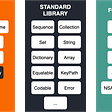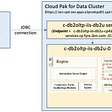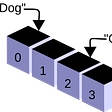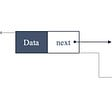# Data Structures and Algorithms

Data Structure can be defined as:

The logic or mathematical model of a particular organization of data is called a data structure

There are many examples of data structures including Arrays, Linked Lists, Stack and Queues.

In interviews questions about Data Structures and Algorithms have been asked to check how much you know about programming.

In this article I’ll briefly explain arrays and linked lists along with their run time complexity.Before explaining data structure, I’m gonna explain Big O Notation.

# Big O notation

According to Wikipedia:

“Big O notation is a mathematical notation that describes the limiting behavior of a function when the argument tends towards a particular value or infinity.”

This looks so difficult to understand. In other words we use Big O to describe the performance of an algorithm. And this helps us to determine whether or not the given algorithm is scalable.

If your code executes completely well on your computer, it does not mean that it performs well when you give it a large data set. This is why we use Big O notation O(n) to describe the performance of an algorithm.

## Why do we learn Big O notation?

In data structures and algorithms, certain operations are more or less costly depending on what data structure we use such as Arrays or Linked Lists.

For example accessing an array element is super fast. Arrays had fixed length. As we continue to add or remove elements from arrays it has to get resized. And this will get costly as the size if the input grows large.

We use another data structure called Linked lists. It grows and shrinks very quickly but accessing a linked list element by its index is very slow. That is why we study big O notation before we study more about data structure.

## Logarithmic Curve vs Linear Curve

If we compare logarithmic curve with linear curve then the linear curve grows at the same rate while the logarithmic curve will slow down at some point. So an algorithm that runs in a logarithmic curve is more efficient and scalable than in a linear curve.

Linear Search Algorithm

Suppose we have an array of sorted numbers from 1 to 10. We have to find the number 10. One way is to find the number by linear search algorithm. We have to iterate over this array using a for loop. Going forward one by one until we find the number 10.

Binary Search Algorithm

Another way to find the number is Binary Search Algorithm. Sometimes the given array is too long. So by using the Binary Search Algorithm we can find the number in less time.

We start by looking at the middle of the array. Find the middle number in an array. If the middle number is smaller then the targeted number then we ignore all the numbers on the left side of the middle number and If the middle number is greater then the targeted number then we ignore all the numbers on the right side of the middle number.

In our case we have to find the number 10 and the middle number is 5. So, now we start looking from 6 to 10. Now we find the middle item which is 8. As 8 is smaller then 10 and is on the left side of 10. So we ignore all the numbers at the left side of 8 and focus on the right side. Now we have only 9 and 10. And we easily find our target. As you see this method requires less time to find the targeted number.

This is logarithmic time. In the logarithmic growth we reduce our work by half in every step.

An algorithm with logarithmic time is more scalable then the one with linear time.

## Space Complexity

Space complexity is a function describing the amount of memory an algorithm takes in terms of the amount of input to the algorithm.

There are times where you have limited space like when you build an app for your small mobile device. For this you have to optimize for the space because scalability is not a big factor. Only one user will use your app at that moment.

# Arrays

“An array, is a data structure consisting of a collection of elements, each identified by at least one array index or key.”

Arrays are static. That is why we have to specify the size of the array. And we won’t be able to change the size of an array later on.

We use arrays to store a list of items sequentially. Such as the lists of numbers or objects. Let’s suppose the list of 5 integers. These integers are stored in memory like this: 10, 20, 30, 40, 50.

As you know that integers take 4 bytes of memory. If we consider the address of the first item in memory is 100 then in this case the address of the second location will be stored at the memory location 104. And similarly third item stores 108 and so on.

Run Time Complexity

The run time complexity of this operation is one O(1). Because the calculation of memory address does not involve any complex logic.

## Insertion

What if we want to add items in an array? Consider the array of 5 integers. To add another item in an array we have to resize the array. For this we allocate a new array and copy all the items from the previous array to the new one. Now we add a 6th item in an array.

The time complexity of this operation is O(n)

## Deletion

Again consider the array of 5 integers, if we want to remove the last item we look up its index and clear the memory. This is the best case scenario. In this case the time complexity is O(1)

But if we want to remove the item from the beginning of the array. First remove the first item and move all the items to the left side to fill the gap. This is more costly. This is why it is known as the worst case scenario. The time complexity of this operation is O(n).

We use linked lists to store a list of objects in a sequence. Linked lists can grow and shrink automatically.

Linked lists consist of a group of nodes in a sequence. Each node holds two pieces of data. One is value and other is address of the next node. In this way each node points to the next node. This is why it is called linked lists because all the nodes are linked together.

We can insert and delete nodes in the linked list. Performing each operation has its own time complexity.

## Insertion

If we want to insert an item in a linked list, we have three ways to insert any item. Such as inserting at the end, at the beginning and at the middle. Each time with different time complexity.

1. At the End = O(1)
2. At the Beginning = O(1)
3. At the Middle = O(n)

## Deletion

Similarly we delete the items in the same way. Delete from the middle, from the end and from the beginning. And the time complexity is given is given below:

1. From the Beginning = O(1)
2. From the End = O(n)
3. From the Middle = O(n)

--

--

--

## More from Salman Maken

I write about Computer Science, Tech and Literature.

Love podcasts or audiobooks? Learn on the go with our new app.

## A leading social communication app uses StarRocks to improve multidimensional monitoring system## DEFIROCK x IM community AMA RECAP## What are the differences between Swift, the standard library and Foundation?## Externalize Database from Information Server using NodePort## Numpy Array Operations## Buddy V’s Cake Slice## FACINGS Improving eospyo Code for Better Backend Blockchain Integration## CXL Institute Digital Analytics Minidegree Review Part 9## Salman Maken

I write about Computer Science, Tech and Literature.

## Arrays Data Structures Explained## Linked lists: what are they and how do they work?